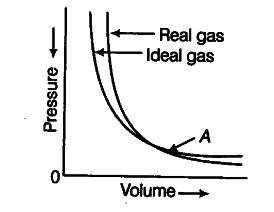# Pressure versus volume graph for a real gas

Pressure versus volume graph for a real gas and an ideal gas are shown in figure. Answer the following questions on the basis of this graph.(i) Interpret the behaviour of real gas with respect to ideal gas at low pressure.
(ii) Interpret the behaviour of real gas with respect to ideal gas at high pressure.
(iii) Mark the pressure and volume by drawing a line at the point where real gas behaves as an
ideal gas.

(i) At low pressure, the real gas shows very small deviation from ideal behaviour because the two curves almost coincide at low pressure.
(ii) At high pressure, the real gas show large deviations from ideal behaviour as the curves are far apart.
. (iii) At point ‘A’, both the curves intersect each other. At this point real gas behaves as an ideal gas. \$p_{ 1 }\$ and \$V_{ 1 }\$ are the pressure and volume which corresponds to this point A.